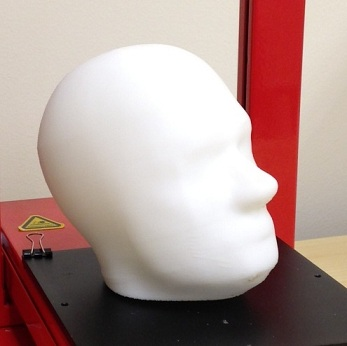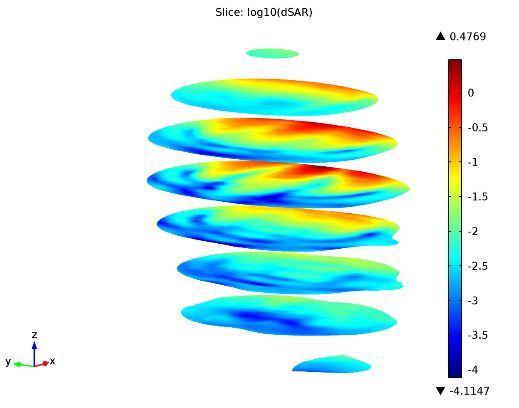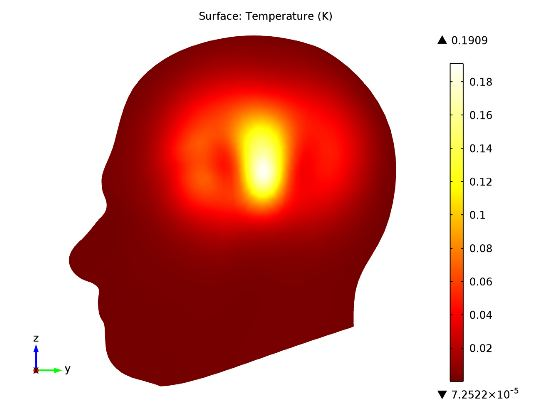# Specific Absorption Rate (SAR) in the Human Brain

August 12, 2013

It seems everyone and their kid brother has a cell phone these days — and we are constantly using them. We don’t just rely on them to make calls anymore, either; they serve as our maps, calendars, to-do lists, channel for social interaction, and so forth. This continuous use begs the question: “What about the radiation our phones emit, and how much of it is absorbed by our brains?” When considering this, scientists use the specific absorption rate (SAR) to learn how much of the radiation is absorbed by human tissue.

### About the Specific Absorption Rate

The specific absorption rate (SAR) is a measurement used for determining the amount of radiation absorbed by human tissue when exposed to a radio frequency (RF) electromagnetic field. What’s referred to as the SAR value is a common property used for measuring absorbed energy, and this value is calculated as:

E_{ \mathsf S \mathsf A \mathsf R}=\sigma{\frac{ \begin{vmatrix} \mathbf E \end{vmatrix}^2}{\rho}}

Here, σ is the human brain tissue’s conductivity, ρ is the density, while the norm of the electric field is represented by \begin{vmatrix} \mathbf E \end{vmatrix}.

The SAR is typically averaged over the entire body or a smaller sample volume of 10 or 1 g of tissue (which one of these sizes the sample is depends on national rules).

### Setting up a Model of the Human Head

What if we wanted to find out how much of the radiation emitted from a cell phone is absorbed by the tissue in the cell phone user’s brain? How does the absorbed radiation affect the temperature in the brain tissue? Both of these questions can be resolved by setting up and modeling the problem in COMSOL Multiphysics along with the RF Module and Heat Transfer Module. For our model, we will use the local SAR value, not the average value, in which case it’s important to note that the maximum local SAR will always be higher than the maximum SAR value.

Before we can get started with our simulation, we need to import a geometry representing the human head. We will use a slightly adjusted version of a geometry provided by IEEE, IEC, and CENELEC based on their standard specification of SAR value measurements (see a print-out of this model below).The human head geometry used to model SAR printed in 3D.

Note that the human head contains a variation of tissue as opposed to being consistent throughout. To account for this, we used data from an MRI of a human head containing 109 slices that are 256-by-256 voxels each. While this exact data has no scientific background, it does serve the purpose of demonstrating a variation in conductivity, permittivity, and perfusion rate as a function of the position inside the head.

### Modeling Local SAR Value in the Human Brain

Assuming the radiation is at a frequency of 835 MHz, we can visualize the local SAR value with a log-scale slice plot:Plot showing local SAR value in the brain tissue.

As you can see above, the SAR value is highest near the head’s surface, where it faces the incident wave. You can also notice the difference in electrical properties via the colors in the log-scale plot.

Next, let’s tackle the temperature aspect of our problem. For this, we will need to use the bioheat equation, which takes heat loss due to blood flow into account. Intuitively, if we plot this, the temperature is highest near the antenna. The brain tissue temperature increases by under 0.2°C (shown in K in the surface plot below), and drops quickly once inside the head.Local increase in temperature just under the cell phone antenna.

### How dangerous is cell phone radiation?

While no one seems to agree on exactly how dangerous cell phone radiation is to the human brain, the general consensus is that it’s a good idea to keep it at low doses. In the meantime, scientists can continue to use the SAR value to determine how much radiation is absorbed by the brain, and simulation software to model these types of scenarios to ensure new designs comply with rules and regulations for radiation emission levels.

#### Categories##### Mohand Alzuhiri
August 25, 2015

i just have one question, as you know the SAR is frequency dependent, why didn’t you mention it in your equations.##### Fanny Littmarck
August 31, 2015##### gisoo barazandeh
July 2, 2017

Hi

I need to remodel the Comsol’s SAR in human head for 1800 MHZ frequency to measure SAR in human head but I face a problem. I use the Comsol model, it works for 800 and 900 MHZ but as for 1800 MHZ it doesn’t converge after 2 days of being computing.This problem exists for frequencies higher than 1400MHZ. can you help?##### Fanny Littmarck
July 3, 2017

Hello Gisoo,##### Cédric AMORIM
October 19, 2017

Hello, how do you calculate the DAS with Comsol?
Do you have a specific formula or are you using a software type Matlab after calculating the SAR in your model?##### Cédric AMORIM
October 19, 2017

Hello, how do you calculate the DAS with Comsol?
Do you have a specific formula or are you using a software type Matlab after calculating the SAR in your model?##### Cédric AMORIM
October 19, 2017

Sorry for the multi-posts, error from my smartphone.
Hello, how do you calculate the SAR10g with Comsol?
Do you have a specific formula or are you using a software type Matlab after calculating the maximum SAR in your model?##### Jiyoun Munn
November 3, 2017

In https://www.comsol.com/model/absorbed-radiation-sar-in-the-human-brain-2190, check the model and its documentation for calculating the SAR value.

EXPLORE COMSOL BLOG